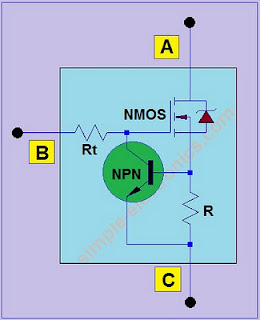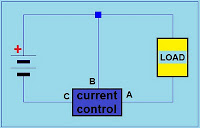### 5Constant Current Source using MOSFET

PNP-PMOS version
This  circuit is a constant current source using PMOS, a resistor, and  PNP bipolar junction transistor. It is very simple to construct and very useful in battery chargers and  other circuits that requires limiting current or constant current sources.PMOS version schematic
You can put this circuit in series to your circuit component which you wish to control its current. See sample circuit below.PMOS connection diagram
Materials:
PNP - General purpose PNP transistor such as C9012, BC557, BC556, and others
PMOS - such as IRF4905 and similar Mosfet
Rt - 10 kilo ohms
R - dependent on the current you need

NPN-NMOS versionNMOS version schematic
Diagram on how it will be connected is shown below.NMOS connection diagram
Materials:
NPN - 9013, 2N2222, or similar transistor
NMOS - IRF530 or similar Mosfet
Rt - 10 kilo ohms
R - dependent on the current you need

For both of the control circuits, the value of current is:
Current  = 0.7/R;  for silicon bjt transistor.
Sample Current vs Resistor values :
7A use 0.1 ohms 5watts
5A use 0.12 ohms 5watts
1.5A use .47 ohms 1watt

1.A resistor has to be grounded through the transistor-collector

2.yeah you are right bapu. tnx, im working on its drawing. Ill add NMOS version also

3.A Zener diode is needed from Gate to Source. It is only needed if this circuit is used in designs where the Voltage to the Load exceeds the rating of the Gate to Source Voltage of the PMOS or NMOS FET. The value of the Zener diode will depend on the Maximum Gate to Source Voltage specified by the manufacturer of the PMOS or NMOS FET.

4.Actually the zener diode in the circuit is part of the Mosfet symbol. In actual circuit construction, no zener diode is required.

5.DH-gm... I do know about the internal zener diode in the Mosfet because of the design of the Mosfet. That zener diode is tied from Source-to-Drain and NOT tied from the Source-to-Gate of the Mosfet.

From experience in a circuit that we designed, We used the PMOS version of what you show in the picture above. The voltage in the circuit was 48V. The Mosfet was destroyed because the voltage from "A" (Source) to "B"(Gate) was 48V which was above the Datasheet specifications of a Gate-to-Source maximum of 20 Volts. The problem was fixed by adding a 15V Zerer Diode from the Source of the Mosfet to the Gate of the Mosfet. This kept the Gate-to-Source voltage clamped at 15V.

From International Rectifier's Datasheet on the IRF4905 (page 1) under ABSOLUTE MAXIMUM RATINGS: Vgs (Gate-to-Source Voltage)= +/-20V. Therefore if the circuit voltage is at or above 20 Volts the Mosfet can sustain permanent damage.